# 力扣 1514——概率最大的路径<!-- more -->

﻿

## 原题

﻿

﻿

﻿

﻿

﻿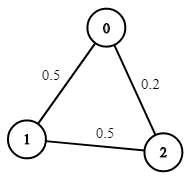﻿

输入：n = 3, edges = [[0,1],[1,2],[0,2]], succProb = [0.5,0.5,0.2], start = 0, end = 2输出：0.25000解释：从起点到终点有两条路径，其中一条的成功概率为 0.2 ，而另一条为 0.5 * 0.5 = 0.25

﻿

﻿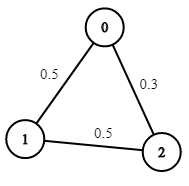﻿

输入：n = 3, edges = [[0,1],[1,2],[0,2]], succProb = [0.5,0.5,0.3], start = 0, end = 2输出：0.30000

﻿

﻿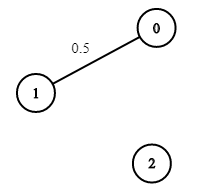﻿

输入：n = 3, edges = [[0,1]], succProb = [0.5], start = 0, end = 2输出：0.00000解释：节点 0 和 节点 2 之间不存在路径

﻿

﻿

• 2 <= n <= 10^4

• 0 <= start, end < n

• start != end

• 0 <= a, b < n

• a != b

• 0 <= succProb.length == edges.length <= 2*10^4

• 0 <= succProb[i] <= 1

• 每两个节点之间最多有一条边

﻿

## 解题

﻿

### 首次尝试

﻿

class Solution {    /**     * key为起始点，value为所有相连的点     */    Map<Integer, Set<Integer>> map;    /**     * key为"点A_点B"(A < B)，value为对应的概率     */    Map<String, Double> probMap;    double maxProb = -1;    int end;    public double maxProbability(int n, int[][] edges, double[] succProb, int start, int end) {        map = new HashMap<>(n * 4 / 3 + 1);        probMap = new HashMap<>(succProb.length * 4 / 3 + 1);        this.end = end;        // 构造每个点的相连关系        for (int i = 0; i < edges.length; i++) {            int[] edge = edges[i];            Set<Integer> set = map.computeIfAbsent(edge, k -> new HashSet<>());            set.add(edge);            set = map.computeIfAbsent(edge, k -> new HashSet<>());            set.add(edge);            String key = edge < edge ? (edge + "_" + edge) : (edge + "_" + edge);            probMap.put(key, succProb[i]);        }        boolean[] visited = new boolean[n];        dp(start, 1, visited);        return maxProb == -1 ? 0 : maxProb;    }    public void dp(int index, double prob, boolean[] visited) {        // 已到终点        if (index == end) {            maxProb = prob > maxProb ? prob : maxProb;            return;        }        // 获取当前点可以到达的所有点        Set<Integer> set = map.get(index);        // 如果当前点到达不了其余点        if (set == null) {            return;        }        // 标记当前点已访问        visited[index] = true;        // 遍历相邻的点        for (int next : set) {            if (visited[next]) {                continue;            }            String key = index < next ? (index + "_" + next) : (next + "_" + index);                        // 访问下一个点            dp(next, prob * probMap.get(key), visited);        }        // 退出，将该点标记为未访问        visited[index] = false;    }}

﻿

﻿

﻿

﻿

﻿

### Dijkstra 算法

﻿

#### 定义概览

﻿

Dijkstra (迪杰斯特拉)算法是典型的单源最短路径算法，用于计算一个节点到其他所有节点的最短路径。主要特点是以起始点为中心向外层层扩展，直到扩展到终点为止。

﻿

注意该算法要求图中不存在负权边。

﻿

﻿

﻿

﻿

﻿

﻿

﻿

#### 算法步骤

﻿

1. 初始时，S 只包含源点，即 S ＝{v}，v 的距离为0。U 包含除 v 外的其他顶点，即: U ={其余顶点}，若 v 与 U 中顶点 u 有边，则<u,v>正常有权值，若u不是v的出边邻接点，则<u,v>权值为∞。

2. 从U中选取一个距离v最小的顶点k，把k，加入S中（该选定的距离就是v到k的最短路径长度）。

3. 以k为新考虑的中间点，修改U中各顶点的距离；若从源点v到顶点u的距离（经过顶点k）比原来距离（不经过顶点k）短，则修改顶点u的距离值，修改后的距离值的顶点k的距离加上边上的权。

4. 重复步骤b和c直到所有顶点都包含在S中。

﻿

﻿﻿

#### 本题解法

﻿

class Solution {    public double maxProbability(int n, int[][] edges, double[] succProb, int start, int end) {        // records[i]代表点i相邻的所有点，以及其概率        List<List<Record>> allRecords = new ArrayList<>(n + 1);        for (int i = 0; i < n + 1; i++) {            allRecords.add(new LinkedList<>());        }        // 构造每个点的相连关系        for (int i = 0; i < edges.length; i++) {            int[] edge = edges[i];            List<Record> records = allRecords.get(edge);            records.add(new Record(edge, succProb[i]));                        records = allRecords.get(edge);            records.add(new Record(edge, succProb[i]));        }        // 利用广度优先搜索，进行遍历        // 借用优先队列，保证优先遍历当前概率高的        PriorityQueue<Record> queue = new PriorityQueue<>();        // 记录从start到每一个点的概率        double[] result = new double[n];        // 从start开始遍历        queue.offer(new Record(start, 1));        result[start] = 1;        // 开始        while (!queue.isEmpty()) {            // 当前节点            Record record = queue.poll();            int node = record.node;            double prob = record.prob;            // 获取当前点所能达到的其他节点            List<Record> otherNodes = allRecords.get(node);            // 遍历其余节点            for (Record next : otherNodes) {                int nextNode = next.node;                double nextProb = prob * next.prob;                // 如果当前计算出的概率，小于等于之前计算的概率                if (nextProb <= result[nextNode]) {                    // 那么就没有必要继续算了，直接用之前的即可                    continue;                }                                // 更新概率                result[nextNode] = nextProb;                // 如果已到结尾或者当前的概率已经比到end的小                if (nextNode == end || nextProb < result[end]) {                    // 那么也没有必要继续了                    continue;                }                // 添加节点                queue.offer(new Record(nextNode, nextProb));            }        }        return result[end];    }    class Record implements Comparable<Record> {        int node;        double prob;        public Record(int node, double prob) {            this.node = node;            this.prob = prob;        }        @Override        public int compareTo(Record other) {            if (other == null) {                return -1;            }            if (this.prob == other.prob) {                return this.node - other.node;            }            return this.prob - other.prob > 0 ? -1 : 1;        }    }}

﻿

﻿

﻿

### Bellman-Ford 算法

﻿

﻿

#### 算法步骤

﻿

1. 创建源顶点 v 到图中所有顶点的距离的集合 distSet，为图中的所有顶点指定一个距离值，初始均为 Infinite，源顶点距离为 0；

2. 计算最短路径，执行 V - 1 次遍历；对于图中的每条边：如果起点 u 的距离 d 加上边的权值 w 小于终点 v 的距离 d，则更新终点 v 的距离值 d；

3. 检测图中是否有负权边形成了环，遍历图中的所有边，计算 u 至 v 的距离，如果对于 v 存在更小的距离，则说明存在环；

﻿

﻿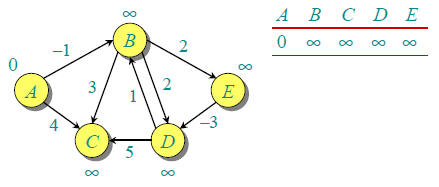﻿

﻿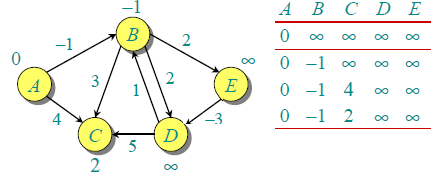﻿

﻿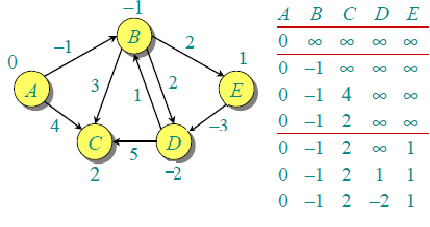﻿

﻿

#### 本题解法

﻿

class Solution {  public double maxProbability(int n, int[][] edges, double[] succProb, int start, int end) {    // 记录结果    double[] result = new double[n];    // 起点    result[start] = 1;    // 从start点出发，先更新直接与start点相连的点的概率，然后逐步更新，直到不需要更新为止    while (true) {      // 是否有过变动      boolean changed = false;      // 遍历所有边      for (int j = 0; j < edges.length; j++) {        int[] edge = edges[j];        // 如果从当前点edge出发，到edge的概率，大于之前记录的结果        if (result[edge] * succProb[j] > result[edge]) {          // 则更新          result[edge] = result[edges[j]] * succProb[j];          changed = true;        }        // 因为是无向图,所以再反向遍历        if (result[edge] * succProb[j] > result[edge]) {          result[edge] = result[edge] * succProb[j];          changed = true;        }      }      // 一遍未修改则表示图已遍历完成      if (!changed) {        break;      }    }    return result[end];  }}

﻿

﻿

## 总结

﻿

﻿

https://death00.github.io/

﻿

﻿﻿﻿## 评论